Courses

# Thick Cylinders Stresses due To Internal And External Pressures Mechanical Engineering Notes | EduRev

## Mechanical Engineering : Thick Cylinders Stresses due To Internal And External Pressures Mechanical Engineering Notes | EduRev

The document Thick Cylinders Stresses due To Internal And External Pressures Mechanical Engineering Notes | EduRev is a part of the Mechanical Engineering Course Machine Design.
All you need of Mechanical Engineering at this link: Mechanical Engineering

Stresses in thick cylinders

For thick cylinders such as guns, pipes to hydraulic presses, high pressure hydraulic pipes the wall thickness is relatively large and the stress variation across the thickness is also significant. In this situation the approach made in the previous section is not suitable. The problem may be solved by considering an axisymmetry about z-axis and solving the differential equations of stress equilibrium in polar co-ordinates. In general the stress equations of equilibrium without body forces can be given as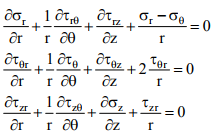(1)

For axisymmetry about z-axis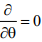and this gives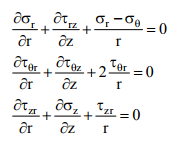(2)

In a plane stress situation if the cylinder ends are free to expand σz = 0 and due to uniform radial deformation and symmetry τrz = τθz = τ = 0. The equation of equilibrium reduces to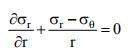This can be written in the following form:       (3)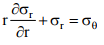If we consider a general case with body forces such as centrifugal forces in the case of a rotating cylinder or disc then the equations reduce to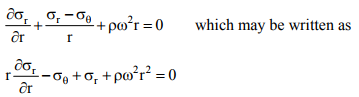(4)

It is convenient to solve the general equation so that a variety of problems may be solved. Now as shown in figure- 9.2.1.1, the strains εr and εθ may be given by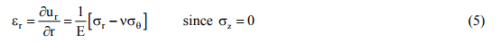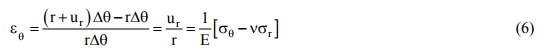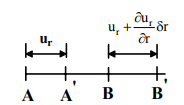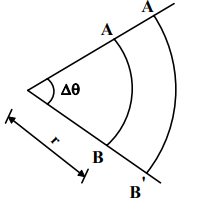9.2.1.1F- Representation of radial and circumferential strain.

Combining equation (5) and (6) we have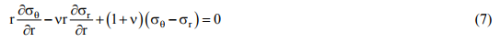Now from equation (4) we may write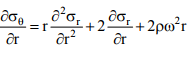and combining this with equation (7) we may arrive at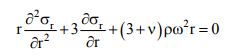(8)

For a non-rotating thick cylinder with internal and external pressures pi and po we substitute ω = 0 in equation (8) and this gives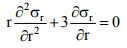(9)

A typical case is shown in figure- 9.2.1.2. A standard solution for equation (9) is σr = crn where c and n are constants. Substituting this in equation (9) and also combining with equation (3) we have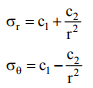(10)

where cand c2 are constants.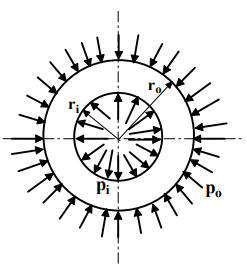9.2.1.2F- A thick cylinder with both external and internal pressure

Boundary conditions for a thick cylinder with internal and external pressures pi and po respectively are:

at r = ri σr = -p
and at r = ro σr = -po

The negative signs appear due to the compressive nature of the pressures. This gives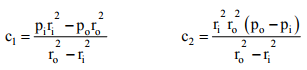The radial stress σr and circumferential stress σθ are now given by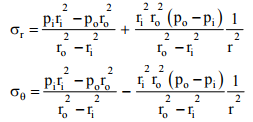(11)

It is important to remember that if σθ works out to be positive, it is tensile and if it is negative, it is compressive whereas σr is always compressive irrespective of its sign. Stress distributions for different conditions may be obtained by simply substituting the relevant values in equation (11). For example, if po = 0 i.e. there is no external pressure the radial and circumferential stress reduce to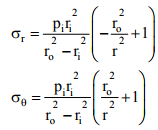(12)

The stress distribution within the cylinder wall is shown in figure- 9.2.1.3.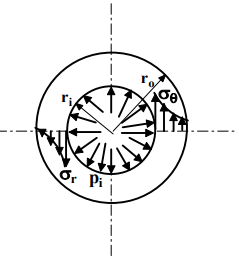9.2.1.3F- Radial and circumferential stress distribution within the cylinder wall when only internal pressure acts.

It may be noted that σr + σθ = constant and hence the deformation in z-direction is uniform. This means that the cross-section perpendicular to the cylinder axis remains plane. Hence the deformation in an element cut out by two adjacent cross-sections does not interfere with the adjacent element. Therefore it is justified to assume a condition of plane stress for an element in section 9.2.1.

If pi = 0 i.e. there is no internal pressure the stresses σr and σθ reduce to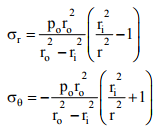(13)
The stress distributions are shown in figure-9.2.1.4.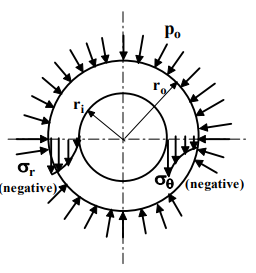9.2.1.4F- Distribution of radial and circumferential stresses within the cylinder wall when only external pressure acts.

Methods of increasing the elastic strength of a thick cylinder by pre-stressing

In thick walled cylinders subjected to internal pressure only it can be seen from equation (12) that the maximum stresses occur at the inside radius and this can be given by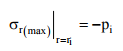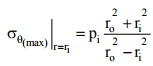This means that as pi increases σθ may exceed yield stress even when p< σyield. Furthermore, it can be shown that for large internal pressures in thick walled cylinders the wall thickness is required to be very large. This is shown schematically in figure 9.2.2.1. This means that the material near the outer edge is not effectively used since the stresses near the outer edge gradually reduce (Refer to figure- 9.2.1.3).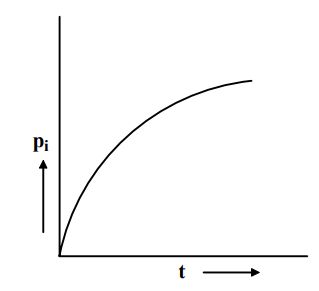9.2.2.1F- A schematic variation of wall thickness with the internal pressure in a thick walled cylinder.

In order to make thick-walled cylinders that resist elastically large internal pressure and make effective use of material at the outer portion of the cylinder the following methods of pre-stressing are used:

1. Shrinking a hollow cylinder over the main cylinder.
2. Multilayered or laminated cylinders.
3. Autofrettage or self hooping.

1. Composite cylinders
An outer cylinder (jacket) with the internal diameter slightly smaller than the outer diameter of the main cylinder is heated and fitted onto the main cylinder. When the assembly cools down to room temperature a composite cylinder is obtained. In this process the main cylinder is subjected to an external pressure leading to a compressive radial stress at the interface. The outer cylinder or the jacket is subjected to an internal pressure leading to a tensile circumferential stress at the inner wall. Under this condition as the internal pressure increases the compression in the inner cylinder is first released and then only the cylinder begins to act in tension. Gun barrels are normally pre-stressed by hooping since very large internal pressures are generated.

Here the main problem is to determine the contact pressure ps. At the contact surface the outer radius rsi of the inner cylinder is slightly larger than the inside diameter rso of the outer cylinder. However for stress calculations we assume that  rso ≌  rsi = rs (say). The inner and outer cylinders are shown in figure- 9.2.2.2.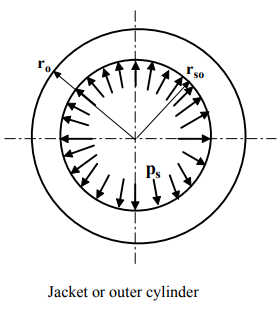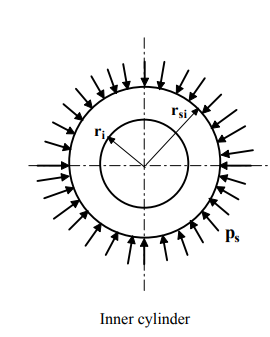9.2.2.2F- Dimensions and the pressures at the contact surface of the internal and outer cylinders.

For the outer cylinder the radial and circumferential stresses at the contact surface may be given by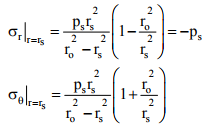In order to find the radial displacements of the cylinder walls at the contact we consider that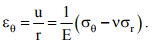This gives the radial displacement of the inner wall of the outer cylinder as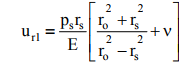Similarly for the inner cylinder the radial and circumferential stresses at the outer wall can be given by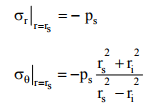And following the above procedure the radial displacement of the contact surface of the inner cylinder is given by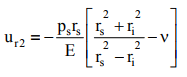The total interference δ at the contact is therefore given by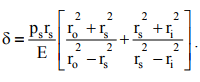This gives the contact pressure in terms of the known variables as follows: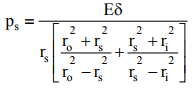The combined stress distribution in a shrink fit composite cylinder is made up of stress distribution in the inner and outer cylinders and this is shown in figure-9.2.2.3.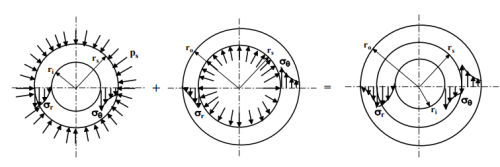9.2.2.3F- Combined stress distribution in a composite cylinder.

Residual circumferential stress is maximum at r = ri for the inner cylinder and is given by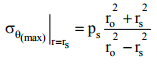Residual circumferential stress is maximum at r = rs for the outer cylinder and is given by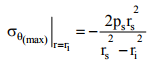Stresses due to fluid pressure must be superimposed on this to find the complete stress distribution.

2. Multilayered or Laminated cylinder

The laminated cylinders are made by stretching the shells in tension and then welding along a longitudinal seam. This is shown in figure- 9.2.2.4.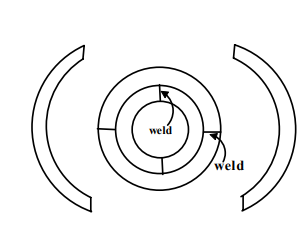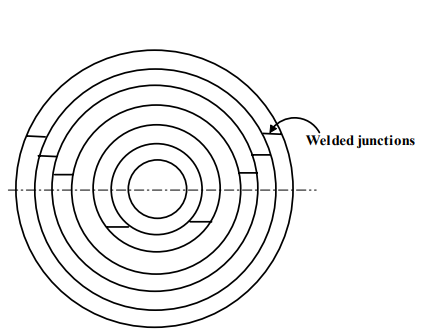9.2.2.4F- Method of construction of multilayered cylinder

3. Autofrettage

In some applications of thick cylinders such as gun barrels no inelastic deformation is permitted. But for some pressure vessel design satisfactory function can be maintained until the inelastic deformation that starts at inner bore spreads completely over the wall thickness. With the increase in fluid pressure yielding would start at the inner bore and then with further increase in fluid pressure yielding would spread outward. If now the pressure is released the outer elastic layer would regain its original size and exert a radial compression on the inner shell and tension on the outer region.

This gives the same effect as that obtained by shrinking a hoop over an inner cylinder. This is known as Self- hooping or Autofrettage. This allows the cylinder to operate at higher fluid pressure. For a given autofrettage fluid pressure a given amount of inelastic deformation is produced and therefore in service the same fluid pressure may be used without causing any additional inelastic deformation.

Offer running on EduRev: Apply code STAYHOME200 to get INR 200 off on our premium plan EduRev Infinity!

## Machine Design

57 videos|71 docs|102 tests

,

,

,

,

,

,

,

,

,

,

,

,

,

,

,

,

,

,

,

,

,

;